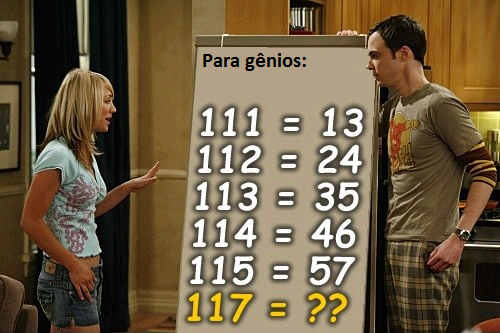# Is 118 Equal to 90, 810, or 8A?

I’m not a mathematician (if you are, please send some tips to improve this post), but I saw an interesting math problem going around the internet and I felt like posting my solution. This the the problem as I first saw it:Everyone seems to have solved this problem looking for a function that relates the two numbers. I actually tried to solve the problem assuming the numbers were actually equal to each other (more on that later), but most people seem to have solved for a function that relates the numbers. Let’s try looking for a function first.

## A simple solution

If you’re looking for a simple pattern, you may notice the numbers on the left are increasing in increments of one, and the numbers on the right by increments of 11. Mind the gap, though, we’re solving for 117 instead of 116. Following this pattern we’ve increased by 2 on the left and should therefore add 22 on the right. So the answer is 79.

This is just a simple linear regression, so you can solve it to find a formula you can use for any number:

There you go, a simple formula that works for all our test cases, and we can easily plug in 12345 and find that the answer with this pattern would be 134587.

## An alternative trick

Some people used an alternative pattern. They took the last digit from the right as the first digit on the left. The remaining digit(s) on the right are the some of the digits on the right. So for 113 the answer starts with 3 (the last number) and ends with 5 (the sum of 1, 1, and 3). Again, this matches all the test cases.

## A different interpretation

I was trying to solve a different problem. I assumed the numbers were actually equal. Of course 111 is not equal to 13 in our familiar decimal numeral system, but programmers are used to working with alternative bases like binary or base 2, octal or base 8 and hexadecimal or base 16.

This is the pattern I found:

```  1112 = 134 = 7
1123 = 245 = 14
1134 = 356 = 23
1145 = 467 = 34
1156 = 578 = 47
```

If you continue this pattern:

```  1167 = 689 = 62
1178 = 7910 = 79
1189 = 8A11 = 98
```

(Note: Once you go past decimal, or base 10, the number system involves letters. So A is the “number” after 9.)

## Comparison

I find it interesting that three pretty simple patterns all work for 7 straight test cases, even though my interpretation solved a different problem! However, they do begin diverging at 118.

 x y (simple solution) y (composition) y (number systems) y (number systems in decimal) 116 68 68 689 62 117 79 79 7910 79 118 90 810 8A11 98

The way the problem is stated is not precise. The popular solutions assume there is an implicit function. The problem could be more precisely stated as:

My solution assumes there are unknown implicit bases. I’m not exactly sure how to state that, but it’s something like this:

```Given:
111f(x) = 13g(x)
112f(x) = 24g(x)
113f(x) = 35g(x)
114f(x) = 46g(x)
115f(x) = 57g(x)

Find:
f(x)
g(x)
f(117) and g(117)
```

I’m curious where this problem originated. Occam’s Razor suggests they had the simple solution in mind, but if the problem is really “for genuises”, then counting by 11 is a bit trivial. It’s too bad they didn’t ask about 118.If the problem was really “for genuises”, then it seems like there’d be a bit more to it than incrementing by 11. They should have asked about 118.